﻿ Computational Analysis of Porous Channel Flow with Cross-DiffusionAmerican Journal of Computational and Applied Mathematics

p-ISSN: 2165-8935    e-ISSN: 2165-8943

2019;  9(5): 119-132

doi:10.5923/j.ajcam.20190905.01### Computational Analysis of Porous Channel Flow with Cross-Diffusion

Chinedu Nwaigwe1, Azubuike Weli1, Oluwole Daniel Makinde2

1Department of Mathematics, Rivers State University, Nigeria

2Faculty of Military Science, Stellenbosch University, South Africa

Correspondence to: Chinedu Nwaigwe, Department of Mathematics, Rivers State University, Nigeria.
 Email: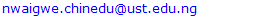Abstract

We investigate the heat and mass transfer in a variable-viscosity channel flow simultaneously accounting for viscous dissipation, external pollutant injection and Soret-Dufour effects. By adopting the Boussinesq approximation and assuming a fully developed uni-direction flow, the set of governing equations are presented. We formulate a finite difference scheme which decouples the system and is amenable to parallel computing. Gauss-Seidel solver is adopted for the resulting linear systems. The numerical results show that the thermal and solutal Grashof numbers, the viscosity parameter and the Darcy number all increase the flow velocity, while the magnetic field and the Prandtl number decrease the flow. It is also observed that the concentration and temperature are opposing each other; an increase in one causes a decrease in the other. Hence, we concluded that a strategy to mitigate pollution in the considered fluid system is to increase the fluid’s temperature.

Keywords: Porous channel flow, Finite difference scheme, Temperature-dependent viscosity, Soret effect, Dufour effects, Viscous dissipation

Cite this paper: Chinedu Nwaigwe, Azubuike Weli, Oluwole Daniel Makinde, Computational Analysis of Porous Channel Flow with Cross-Diffusion, American Journal of Computational and Applied Mathematics , Vol. 9 No. 5, 2019, pp. 119-132. doi: 10.5923/j.ajcam.20190905.01.

### 1. Introduction

Flows through porous media have many applications such as in catalytic converters, fischer-tropsch synthesis, oil reservoirs and bioheat [1-4]. For instance, ground flows have many applications like in oil recovery and energy conversion . Other application areas include packed-bed chemical reactors, gas turbines and material processing facilities .
These applications have led to enormous research in the flow and transport phenomena taking place in porous materials. Studies have been conducted on automobile exhaust systems , Fischer-Tropsch synthesis , in transpiration cooling , and in human tissues . Heat transfer in porous ground is investigated in , see also [11,12] for coupled transport studies in porous media. Bhargava et al.  investigated combined heat and mass transfer in a porous medium, taking into account mass fluxes due to temperature gradients (known as Soret effect) and also heat fluxes due to concentration gradients (known as Dufour effect). Here, we use the term cross-diffusion to refer to the combined presence of both Soret and Dufour effects, see also page 62 in .
Mehmood and Ali  investigated slip condition effects on a viscous channel flow, and the study is extended to the combined effects of both heat injection and suction in . The results show that the flow velocity is enhanced by increase in both the Darcy number and thermal Grashof’s number. Also, the oscillatory flow of a temperature-dependent viscosity Jeffrey fluid in a porous channel is investigated in . They assumed no- slip conditions, incorporated Soret-Dufour effects, an analytical solution is obtained using the homotopy perturbation method. Their results show that the magnetic field decreases the fluid velocity. Two points to note about their work are (i) a time and space dependent problem, as posed by them, requires both boundary and initial conditions. But their initial conditions were not stated, and (ii) they represented the viscous term in the velocity equation as a product of the variable viscosity and the second derivative of the velocity. This is obviously faulty and can only be true if the viscosity were to be a constant. The variable viscosity should appear under the derivative, see [18,6,19,20] for example.
In , the steady heat and mass transfer in a Casson fluid flow over a porous sheet is considered with cross-diffusion. They observed that the Casson parameter decreases skin friction. In an earlier study, Makinde and Mhone  considered the magnetohydrodynamic flow and heat transfer in a channel filled with porous material, see also  for a steady flow. Seth and collaborators  investigated the effects of Joule heating, viscous dissipation, Newtonian heating and thermo-diffussion on the MHD flow of a Casson fluid through a porous medium, see also . The numerical challenges encountered in the presence of an exponentially time-dependent suction have been investigate in [26,27].
Finite volume analysis of heat transfer in the flow of an electrically conducting fluid in a cylindrical domain is studied in , see also  for investigations in cylindrical annulus. In  the channel flow of copper-kerosene nanofluid with magnetic field and stretching wall effects is investigated. Natural convection heat transfer with heat sources in a cylindrical annulus is considered in  while the homotopy analysis of MHD flow between two porous plates intersecting at an angle is studied in . In another study, the Maxwell convective heat transfer model is coupled with the Navier-Stokes fluid model to investigate the flow and thermal characteristics of Titania nanofluids using different base fluids . This set of studies which were conducted by Mebarek-Oudina and collaborators did not consider mass transfer.
In another series of investigations, Makinde and collaborators [34,35] have investigated either heat transfer or mass transfer, but not both, in different geometries; Kumaresan and co-authors  considered the combined effects of heat and mass transfer in a Newtonian fluid, and derived an exact solution using Laplace transform approach, for constant-velocity fluids in porous media. Then, Nwaigwe  extended the study to incorporate combined heat and mass transfer in a concentration-dependent viscosity flow with concentration-dependent diffusivity. One of the cardinal points in  was how to correctly solve the posed problem, hence a sequential implicit scheme was formulated and compared with a Matlab subroutine.
Prasad and collaborators  investigated the heat transfer and porous media flow of a Casson fluid with temperature-dependent thermal conductivity. The steady flow and mass transfer in a porous solid is considered in , and extended to the unsteady case in  without heat transfer; then to the unsteady, temperature-dependent viscosity, non-isothermal case is considered in  without mass transfer. Sharma and coworkers in recent study  further extended theses studies to investigate the influence of both heat and mas transfer in the porous media flow of a Newtonian fluid incorporating Soret effects.
From the above account, it becomes obvious that a full incorporation of heat and mass transfer of variable viscosity fluids with Soret-Dufour effects and pollutant injection is still not complete. In particular, as stated above, the very much commendable work of Selvi and Muthuraji  is incomplete because of the formulation of the viscous term and non specification of initial flow fields; their problem is thus not closed. Moreover, the study did not consider pollutant injection. Secondly, the other impressive work of Sharma and colleagues  only considered constant viscosity fluids, yet Dufour effects are neglected. Moreover, both of these studies adopted analytical methods of solution - the Homotopy perturbation and the Laplace transform methods - which are limited in terms of re-usability and extendability, unlike numerical methods. In this study, we extend the work of  by incorporating variable viscosity and Soret-Dufour effects, while we improve some aspects of the work of Selvi and Muthuraji  by correctly representing the viscous term, incorporating pollutant injection and posing a correct set of initial flow fields consistent with the prescribed boundary conditions. In addition, we propose a numerical scheme which can be easily extended to-and be re-used for other complicated problems.
The remainder of the paper is organized as follows: we present the model equations, including the non-dimensional forms, in section 2. The numerical scheme is formulated in section 3. The numerical results are presented and discussed in section 4 and the paper is concluded in section 5.

### 2. Formulation of the Mathematical Problem

We consider a vertical channel filled with a saturated porous material and bounded by two impermeable walls separated by a distance h, see figure 1. The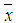and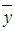axis are as indicated in the figure. We then consider a flow strictly along the channel axis, fully developed and driven by constant pressure gradient and gravitational and magnetic body forces. The two channel walls are immovable and the fluid is incompressible with temperature-dependent viscosity. A uniform magnetic field is applied perpendicular to the walls and viscous dissipation is incorporated.Figure 1. Physical set-up of flow in a saturated vertical porous channel
Further, we assume that the fluid is non-isothermal and some substance is being introduced into the fluid at the rate of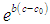per unit volume of the fluid, where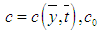and b are, respectively, the substance’s concentration at point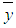and at time,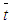; a reference concentration; and a constant. We also incorporate cross-diffusion effects, meaning that combined temperature variations due to mass diffusion and concentration variations due to heat diffusion - the Soret-Dufour effects. Bousinesq’s approximation is adopted for the fluid body forces due to solutal and thermal variations.
On the channel wall boundaries, the no-slip condition apply, and the temperature and concentration are kept constant at Tw and cw respectively. At initial time, the fluid velocity has a parabolic profile with maximum value at the channel center and vanishes at the walls in line with the no-slip conditions. Both the temperature and concentration are initially zero within the fluid except at the walls where the constant values are maintained.
Denoting by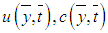and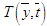the velocity, concentration and temperature, respectively, at point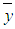and time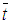; and defining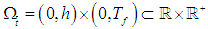. Then, the problem is governed by the following system [18,6,19,20]: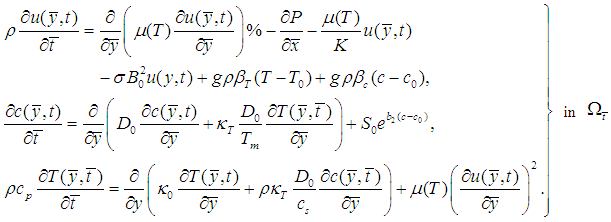(1)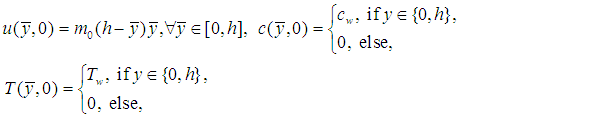(2)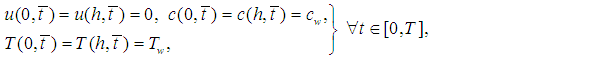(3)
where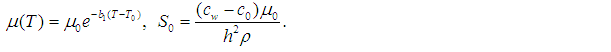(4)
Here, ρ = fluid density,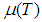= temperature-dependent fluid viscosity, P = fluid pressure, K = permeability of porous medium, σ = electrical conductivity, B0 = uniform magnetic field intensity, g = acceleration due to gravity, βT = temperature expansion coefficient, βC = concentration expansion coefficient, T0 = reference temperature, D0 = diffusion coefficient,= thermal conductivity,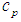= specific heat capacity,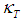= thermal diffusion ratio, m0 = a constant, Tm = mean fluid temperature,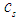= the concentration susceptibility, while b1 and b2 are constants.

#### 2.1. Non-dimensional Formulation

We define the following dimensionless quantities [18,20,6,19]: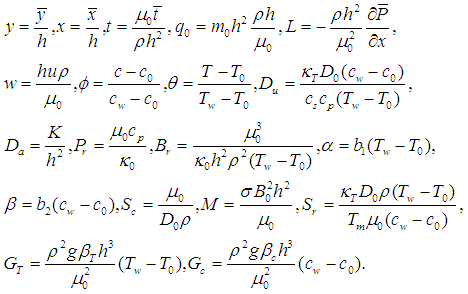(5)
Substituting the nondimensional parameters and variables in (5) into (1)-(4), we obtain the following non-dimensional equations: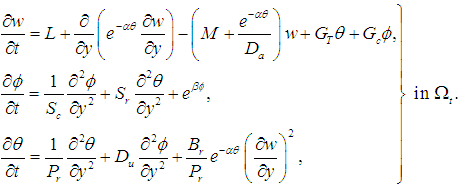(6)
subject to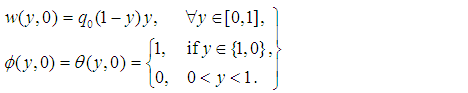(7)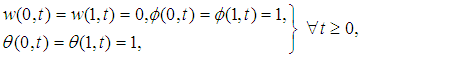(8)
where L = pressure-gradient parameter, α = viscosity parameter, M = magnetic field parameter, Da = Darcy number, GT = Thermal Grashofs number, Gc = solutal Grashofs number, Sc = Schmidt number, Sr = Soret coefficient, β = injection paramter, Pr = Prandtl number, Du = Dufour coefficient, Br = Brinkman number and q0 is a constant [18,6,19].

### 3. Sem-Implicit Numerical Scheme

Let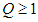be an integer and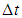be given. Define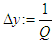;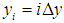for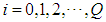;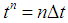for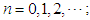and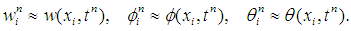Also, define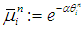, and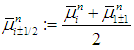. We propose to discretize the coupling and nonlinear source terms explicitly, while the variable-coefficient viscous terms are treated in conservative form. This leads to the following semi-implicit scheme [40,41] see also :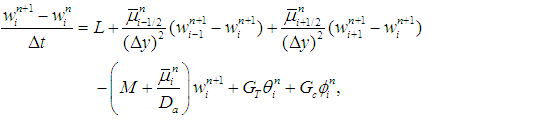(9)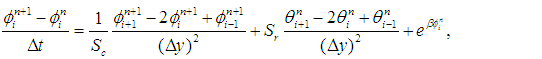(10)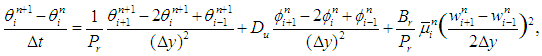(11)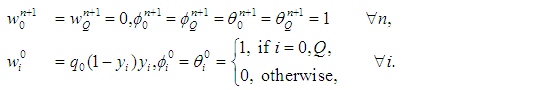(12)
Treating the variable coefficient viscous term in this manner allows to avoid derivatives, which may actually not exist; it also reduce the computational cost. The resulting scheme is decoupled and the solution of each state variable can be computed independently, which in turn allows to leverage parallel computing architectures.

### 4. Numerical Experiments and Results

The numerical results are now presented and discussed. The numerical scheme, (9) - (12), is implemented in an in-house C++ code developed by the first author. We use the following data for all the computations, except when otherwise stated: L = 1.0, α = 0.1, M = 0.3, Da = 0.1, GT = 0.1, Gc = 0.1, Sc = 0.24, Sr = 0.6 β = 0.1, Pr = 0.75, Du = 0.03, Br = 0.1, ∆t = 0.005, q0 = 0.5 and the total duration of simulation is T = 0.5 seconds. The variation of the velocity, concentration and temperature with the flow parameters are considered. The discussion starts with the viscosity parameter α and end with the magnetic field parameter M.
The variation of flow fields with the viscosity parameter α, is shown in figure 2. It is observed that increasing α, which signifies decreasing the fluid viscosity, leads to increase in the flow velocity. The parameter has no effect on the temperature or the concentration.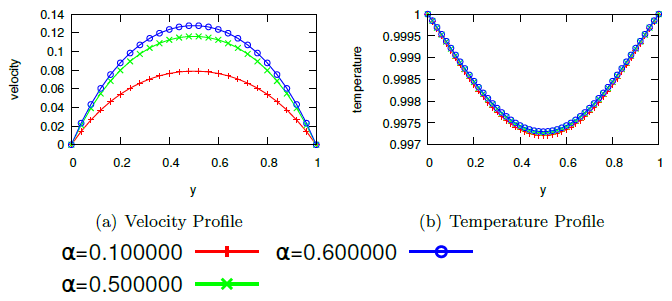Figure 2. Effect of viscosity parameter α on velocity
The effects of the injection parameter β is displayed in figure 3. We can see that increase in β causes an increase in the concentration and a decrease in the temperature. This is obvious because increase in β means addition of the pollutant into the system which should naturally increase the concentration. In this study we observed that increase in concentration is associated with a decrease in the temperature and vice-versa. This is due to cross-diffusion between the concentration and temperature - where one quantity contributes to the flux of the other. Hence, an increase of one leads to non-increase of the other.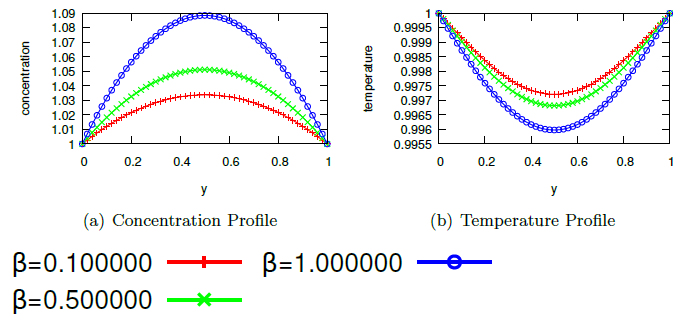Figure 3. Effect of pollutant injection parameter β on the flow Fields
An increase in temperature and a slight decrease in concentration are observed in figure 4. This is because an increase in Br implies an increase in the viscous dissipation. This leads to increased temperature which in turn causes a decrease in the concentration as a result of cross-diffusion. No significant change in velocity is observed and this is due to the opposing effects of the increased temperature and decreased concentration.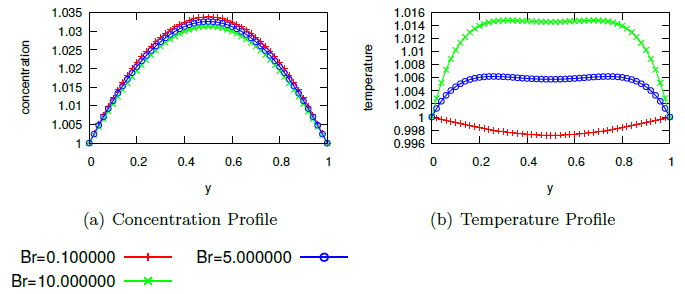Figure 4. Effect of Brinkman number Br on the flow fields
The effect of Darcy number are shown in figure 5 which depicts an increase in velocity and temperature. This is because increasing Darcy number implies to increase the porosity of the medium which should naturally increase the flow, hence the observed increase in velocity. Also, since velocity increase results to increase in viscous dissipation which in turn increases the temperature, hence the observed increase in temperature.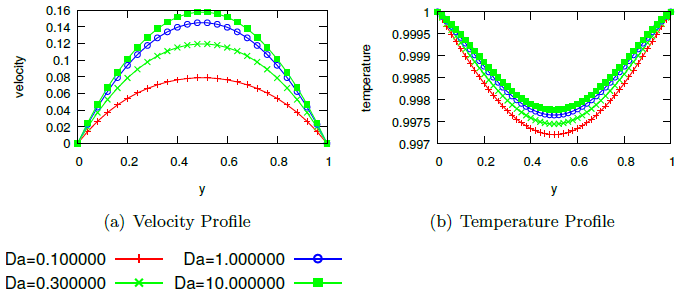Figure 5. Effect of Darcy number Da on the flow fields
The impact of the Prandtl number is displayed in figure 6. As it can be seen, increasing Pr leads to decrease in temperature and velocity but to an increase in concentration. This is because increasing this parameter means to reduce the fluid’s thermal conductivity which reduces the rate at which heat is transported from the heated channel walls into the fluid which was initially at zero temperature. The temperature increase then leads to a reduction in the fluid viscosity hence causing a rise in the velocity. The concentration is increased due to the decrease in temperature - the cross-diffusion effect.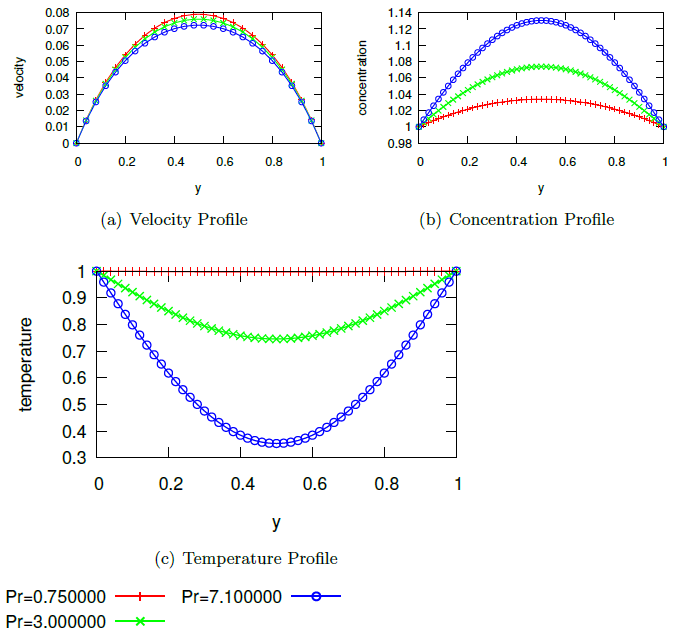Figure 6. Effect of Prandtl number Pr on the flow fields
The effect of Soret number is shown in figure 7. Increase in Sr decreases the temperature but increases the concentration. The Soret effect acts as a source to the pollutant’s concentration leading to increase in the concentration which inturn reduces the temperature due to cross-diffusion. Exactly the same effect is observed in figure 8 in which the Dufour number enhances the concentration but decreases the temperature. This shows that the Soret-Dufour effects seem to favor the concentration but diminish the temperature. The two parameters, Sr and Du did not show any influence on the velocity.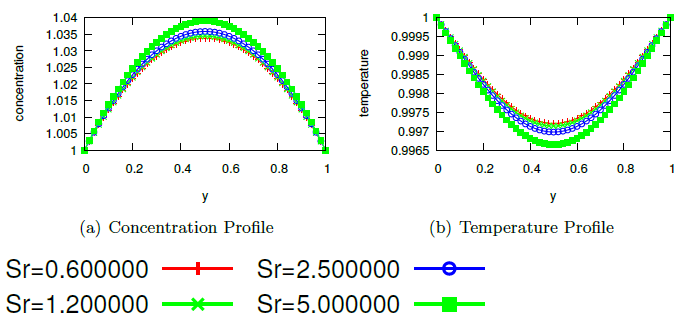Figure 7. Effect of Soret number Sr on the flow fields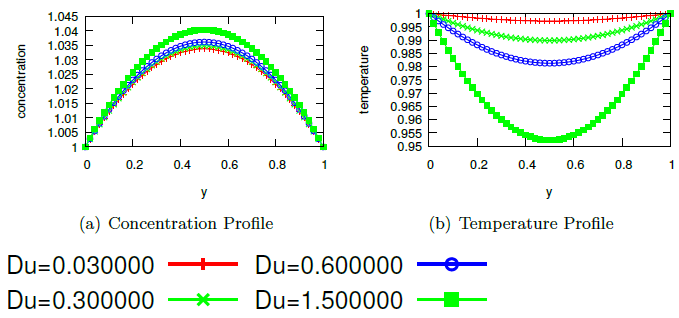Figure 8. Effect of Dufour number Du on the flow fields
The effects of the solutal and thermal Grashof numbers Gc and GT are displayed in figures 9-10. It is observed that both parameters increase the velocity and temperature. This is expected since an increase in Gc and GT means increasing the body force on the fluid which enhances the velocity, and this in turn increases the viscous dissipation, hence increasing the temperature. There is also a very small (insignificant) decrease in concentration, and this obviously due to the cross-diffusion effect - the small temperature increase caused the very small decrease in concentration see figures 9(b) and 10(b).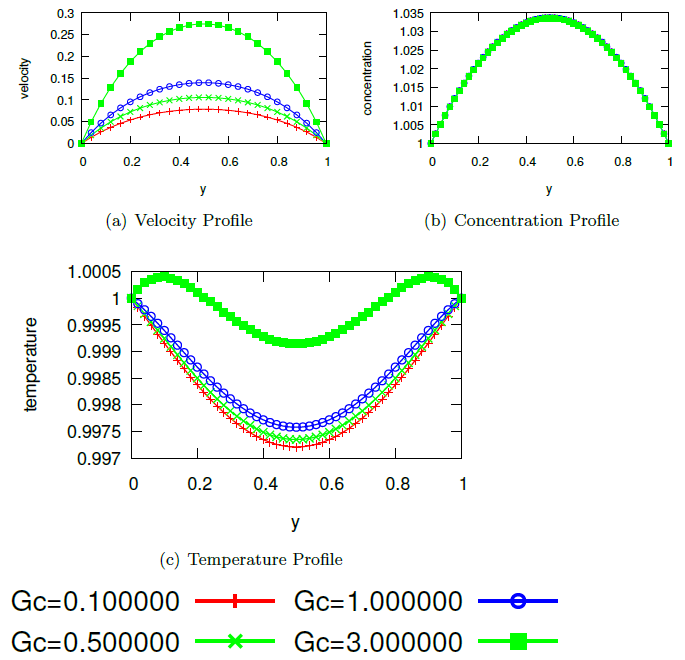Figure 9. Effect of Solutal Grashof number Gc on the flow fields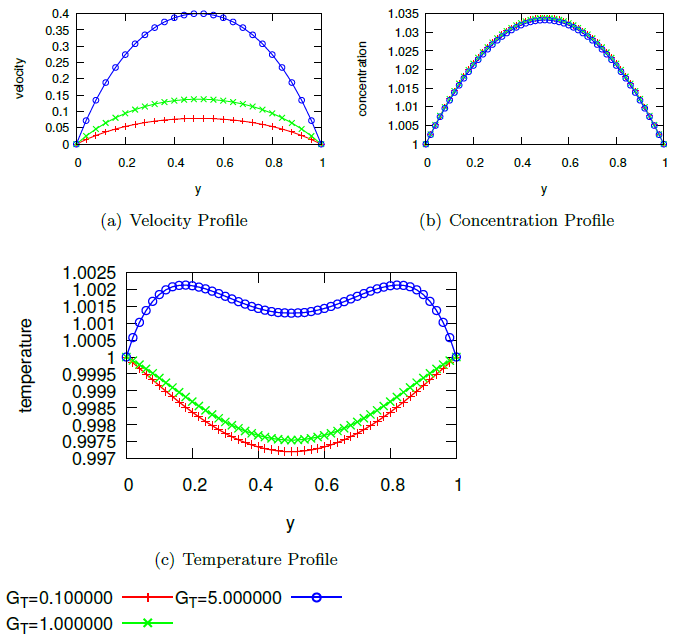Figure 10. Effect of Thermal Grashof number GT on the flow fields
The variations of flow fields with Schmidt number is shown in figure 11. We can see that increasing Sc causes a rise in concentration and a decrease in temperature; no significant change in velocity is observed. This is because, an increase in Sr means to reduce the pollutant diffusivity. Since there is a pollutant injection into the fluid, higher diffusivity will reduce the concentration, while less diffusivity will enhance it. This is why increasing Sr (or reducing diffusivity) increases the concentration. As we noted above, cross-diffusion causes the temperature to decrease. Figure 12 shows that the magnetic field parameter decreases the velocity and have no effect on the concentration or temperature.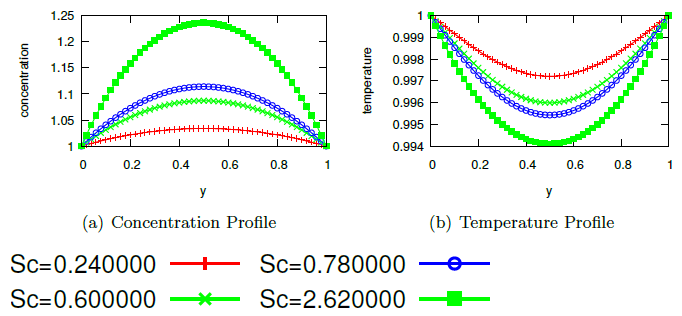Figure 11. Effect of Schmidt number Sc on the flow fields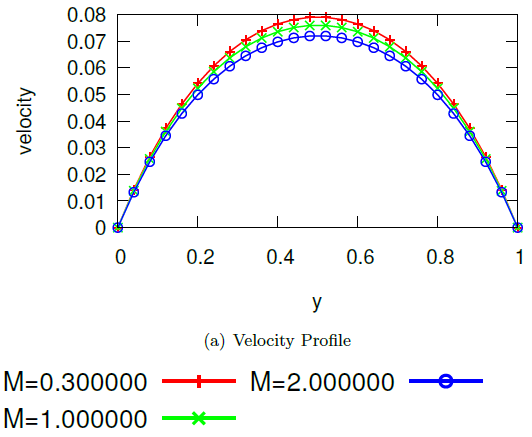Figure 12. Effect of Magnetic field parameter M on the flow fields
The variations of of the skin friction are displayed in figures 13 and 14. It is shown that the skin friction increases with increase in viscosity parameter α, the Darcy number Da, the solutal Grashof’s number Gc and the thermal Grashof’s number GT. It also shown that the skin friction decreases with increasing magnetic field parameter M and the Prandtl number Pr.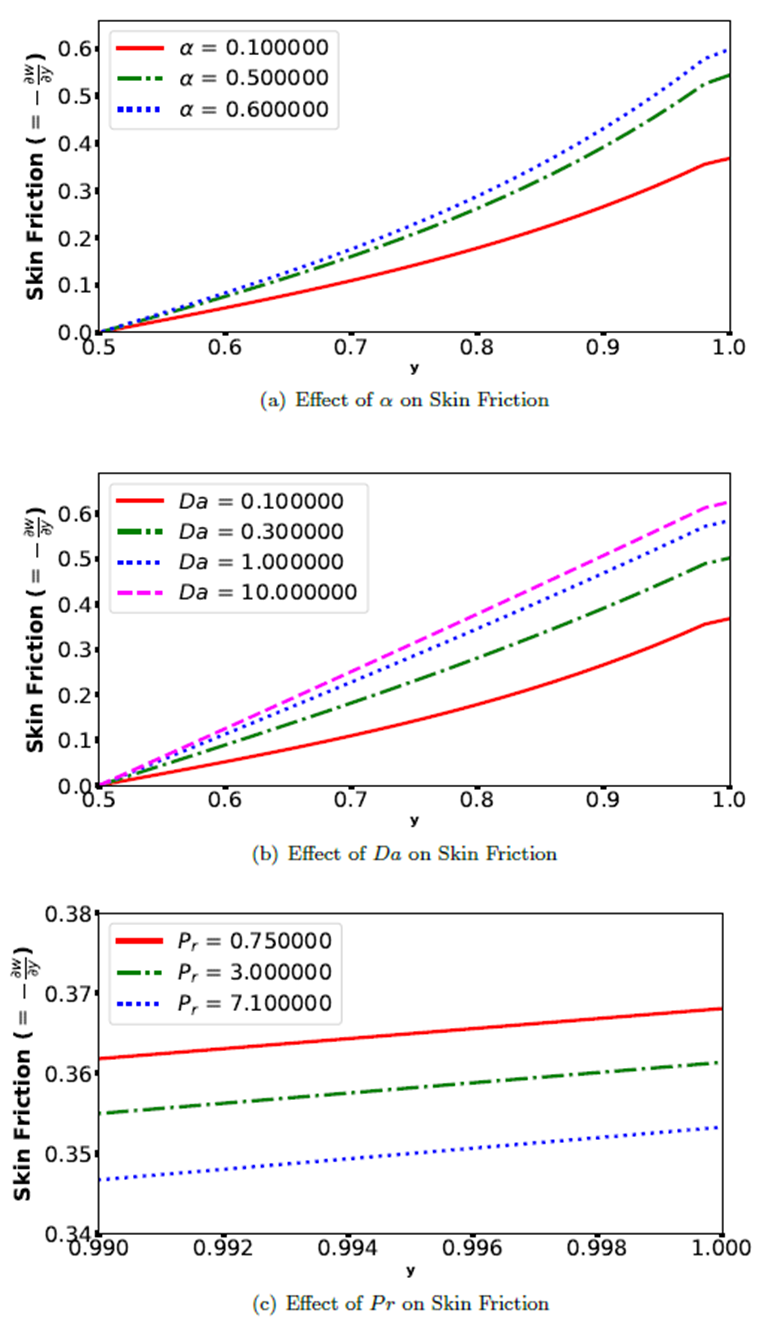Figure 13. Variation of Skin Friction with α, Da and Pr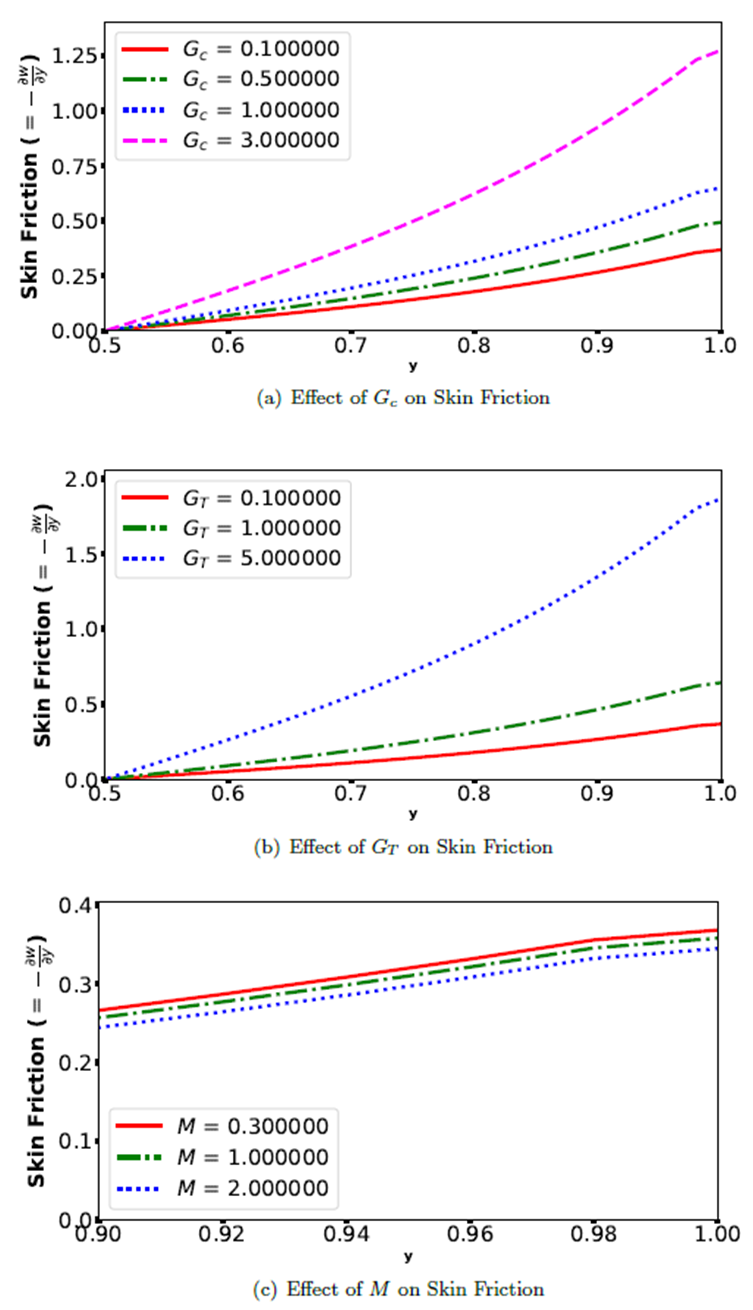Figure 14. Variation of Skin Friction with Gc, GT and M

### 5. Conclusions

This study presented a heat and mass transfer problem in the flow of a temperature dependent viscosity fluid in a saturated porous channel. The effects of dissipation, pollutant injection and cross diffusion are incorporated and the governing equations are solved using a convergent finite difference algorithm. The numerical results show that the flow parameters have opposite effects on the concentration and temperature, meaning that a decrease in one of the two is associated with an increase in the other. We conclude that to eliminate pollutant from the fluid, an increase in temperature might be useful.

### ACKNOWLEDGEMENTS

We are grateful to the reviewers for their beneficial inputs and suggestions. The first author thanks the Petroleum Technology Development Fund (PTDF), Nigeria for funding his PhD at the University of Warwick, UK during 2012-2016.